## 9.4.4 Functional Names

Functions such as log and tan can't simply be typed in as log or tan otherwise they will come out looking like the variables l times o times g () or t times a times n (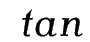). Instead you should use one of the commands listed in Table 9.5. The functions denoted with can have limits by using the subscript command _ or the superscript command ^[Sub- and superscript positioning for operators]. In addition, the modulo commands listed in Table 9.6 are also available.

 \arccos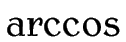\arcsin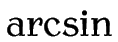\arctan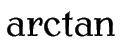\arg\cos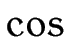\cosh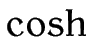\cot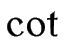\coth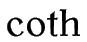\csc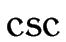\deg\det†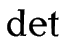\dim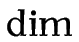\exp\gcd†\hom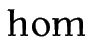\inf†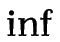\injlim†‡\ker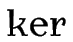\lg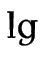\lim†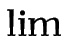\liminf†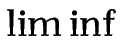\limsup†\ln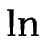\log\max†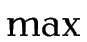\min†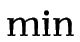\Pr†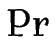\projlim†‡\sec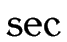\sin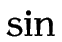\sinh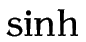\sup†\tan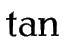\tanh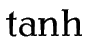\varinjlim†‡\varliminf†‡\varlimsup†‡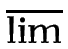\varprojlim†‡Example Input Example Output Command \bmod $m \bmod n$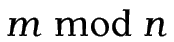\pmod{} $m \pmod{n}$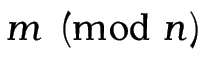\mod{}‡ $m \mod{n}$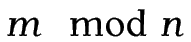\pod{}‡ $m \pod{n}$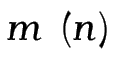Example (Trigonometric Functions):

This example uses the cos and sin functions and also the Greek letter theta.Example (Limit):

The command \infty is the infinity symbol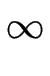, and the command \to displays an arrow pointing to the right. Note the use of _ since the limit is a subscript.The operators with limits behave differently depending on whether they are in displayed or in-line maths. Notice the difference when the same code appears in-line:

In a line of text $\lim_{x\to\infty} f(x)$

which now displays as: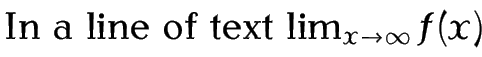Example (With Subscript):

This is another example of a functional name using a subscript:

$\min_x f(x)$Again, notice the difference when it is used in-line:

In a line of text $\min_x f(x)$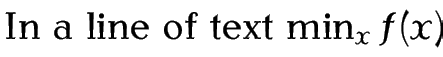### 9.4.4.1 Defining New Functional Operators

It may be that you want a function that isn't specified in Table 9.5. In this case, the amsmath provides the preamble only command

\DeclareMathOperator{<cmd>}{<operator name>}

or its starred variant

\DeclareMathOperator*{<cmd>}{<operator name>}

[Defining a new log-like function in LaTeX]Both versions define a command called <cmd>, which must start with a backslash, that typesets <operator name> as a function name. The starred version is for function names that can take limits (like \lim and \min described above).

Example (Operator Without Limits):

Suppose I want a function called card, which represents the cardinality of a set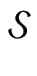. First I need to define the new operator command (which I'm going to call \card) in the preamble:

\DeclareMathOperator{\card}{card}

This operator doesn't take any limits, so I have used the unstarred version.

Later in the document, I can use this new operator command:

$n = \card(\mathcal{S})$In this example \mathcal is used as sets are typically represented in a calligraphic font.

Example (Operator With Limits):

Suppose I now want a function called mode, which represents the mode of a set of numbers. First, I define the operator command in the preamble:

\DeclareMathOperator*{\mode}{mode}

This operator needs to be able to have a subscript, so I have used the starred version.

Later in the document, I can use this new operator command:

$x_m = \mode_{x \in \mathcal{S}}(x)$This book is also available as A4 PDF or 12.8cm x 9.6cm PDF or paperback (ISBN 978-1-909440-00-5).

© 2012 Dickimaw Books. "Dickimaw", "Dickimaw Books" and the Dickimaw parrot logo are trademarks. The Dickimaw parrot was painted by Magdalene Pritchett.